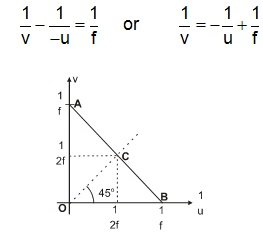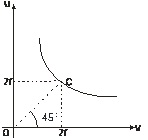# Determination of Focal Length of Convex Lens using u–v Method

The relation between u, v and f for a convex lens is,

v-1 + u-1 = f-1

Using the proper sign convention, u is negative, u negative, v and f are positive. So we have,Comparing with y = mx + c, graph between v-1 & u-1  is negative with slope –1 and intercept  f-1 .The corresponding graph is as shown in figure.

Proceeding in the similar manner as discussed in case of a concave mirror the focal length of the lens can be calculated by measuring the coordinates of either of the points A, B and C.u–v Graph
The u versus v graph is a hyperbola as shown in figure. By measuring the coordinates of point C whose coordinates are (2f, 2f) we can calculate the focal length of the lens.

To read more, Buy study materials of Ray Optics and Optical Instruments comprising study notes, revision notes, video lectures, previous year solved questions etc. Also browse for more study materials on Physics here.